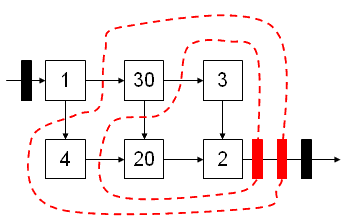# Pipelining Interview Question

Pipe-lining is particular form of re timing where the goal is to increase the throughput (number of results per second) of a circuit. Consider the circuit diagram below; the solid rectangles represent registers, the square are blocks of combinatorial logic:Each combinatorial block in the diagram is annotated with it's propagation delay in ns. For this problem assume that the registers are "ideal", i.e., they have zero propagation delay, and zero setup and hold times.
1. What are the latency and throughput of the circuit above? Latency is how long it takes for a value in the input register to be processed and the result appear at the output register. Throughput is the number of results per second.
2. Pipeline the circuit above for maximum throughput. Pipelining adds additional registers to a circuit; we'll do this by adding additional registers at the output and then use the retiming transformation to move them between the combinational blocks. What are the latency and throughput of your resulting circuit?
Solution:

1. The register-to-register TPD is the TPD of the longest path (timewise) through the combinational logic = 53ns. A value in the input register is processed in one clock cycle (latency = 53ns), and the circuit can produce an output every cycle (throughput = 1 answer/53ns).
2. To maximize the throughput, we need to get the "30" block into it's own pipeline stage. So we'll draw the retiming contours like so:Note there is an alternative way we could have drawn the contours to reach the goal of isolating the "30" block; it might be that other implementation considerations would help pick which alternative was more attracive. Similarly, we could have instead added registers at the input and used retiming to move them into the circuit.
The contours above lead to the following piplelined circuit diagram:A good check to see if the retiming is correct is to verify that there are the same number of registers on every path from the input(s) to the output(s).
The register-to-register TPD is now 30ns, so the throughput of the piplined circuit is 1/30ns. The latency has increased to 3 clock cycles, or 90ns. In general increasing the throughput through pipelining always leads to an increase in latency. Fortunately latency is not an issue for many digital processing circuits -- eg, microprocessors -- where we care more about how many results we get per second much more than how long it takes to process an individual result.